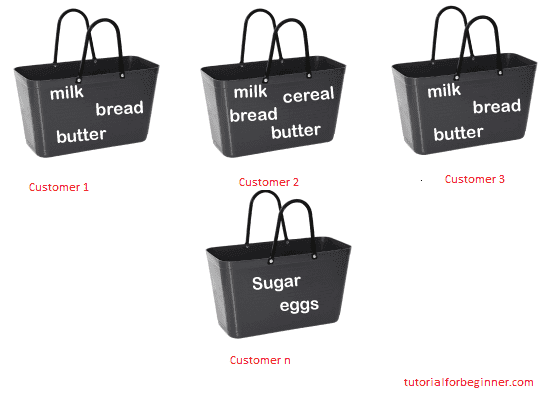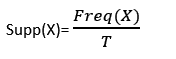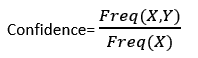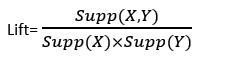# Association Rule Learning in Machine Learning

In this page we will learn Association Rule Learning in Machine Learning, What is Association Rule Learning in Machine Learning?, How does Association Rule Learning work?, Applications of Association Rule Learning.

## What is Association Rule Learning in Machine Learning?

Association rule learning is an unsupervised learning technique that examines the dependency of one data item on another and maps accordingly to make it more profitable. It tries to discover some interesting relationships or links between the dataset's variables. It uses a set of rules to find interesting relationships between variables in a database.

One of the most important topics in machine learning is association rule learning, which is used in Market Basket analysis, Web usage mining, continuous production, and other applications. Market basket analysis is a method used by many large retailers to find the relationships between items. We may explain it by using the example of a supermarket, where all things purchased at the same time are grouped together.

For example, if a consumer buys bread, he'll almost certainly also buy butter, eggs, or milk, therefore these items are kept on the same shelf or in close proximity. Consider the diagram below:Association rule learning can be divided into three types of algorithms:

• Apriori
• Eclat
• F-P Growth Algorithm

We will understand these algorithms in later chapters

## How does Association Rule Learning work?

Association rule learning works on the concept of If and Else Statement, such as if A then B.If element is referred to as the antecedent, and the Then statement is referred to as the Consequent. Single cardinality refers to relationships in which we can discover an association or relationship between two objects. It's all about making rules, and as the number of items grows, so does cardinality. There are numerous metrics for measuring the relationships between thousands of data pieces. These figures are as follows:

• Support
• Confidence
• Lift

Let's understand each of them:

## Support

The frequency of A, or how frequently an item appears in the dataset, is called support. It's the percentage of the transaction T that has the itemset X in it. If there are X datasets, the following can be written per transaction T:## Confidence

The level of confidence represents how often the rule has been proven correct. Or, since the incidence of X is already known, how frequently the elements X and Y appear together in the dataset. It's the ratio of the number of records that contain X to the number of transactions that contain X.## Lift

It is the strength of any rule, which can be defined as below formula:If X and Y are independent of one another, it is the ratio of observed support to expected support. It can take one of three forms:

If X and Y are independent of one another, it is the ratio of observed support to expected support. It can take one of three forms:

• If Lift = 1 : the chances of the antecedent and consequent occurring are independent of one another.
• Lift > 1 : This specifies the degree to which the two itemsets are interdependent.
• Lift < 1 : It indicates that one object can be used in place of another, implying that one item has a negative impact on another.

Types of Association Rule Lerning

Association rule learning can be divided into 3 algorithms:

## Apriori Algorithm

To build association rules, this technique employs a large number of datasets. It's made to deal with databases that have transactions in them. To calculate the itemset efficiently, this algorithm uses a breadth-first search and a Hash Tree.

It is mostly used for market basket analysis and assists in determining which products can be purchased together. It can also be utilized to discover drug reactions in patients in the healthcare field.

## Eclat Algorithm

Equivalence Class Transformation is the name of the Eclat algorithm. This algorithm finds frequent itemsets in a transaction database by using a depth-first search technique. It executes faster than the Apriori Algorithm.

## F-P Growth Algorithm

The F-P growth algorithm is an upgraded variant of the Apriori Algorithm. It stands for Frequent Pattern. It represents the database as a frequent pattern or tree, which is a type of tree structure. This frequent tree's goal is to extract the most common patterns.

## Applications of Association Rule Learning

It can be used in a variety of machine learning and data mining applications. The following are some of the most common uses of association rule learning:

• Market Basket Analysis: One of the most well-known examples and applications of association rule mining is market basket analysis. Big merchants frequently employ this strategy to determine the relationship between items.
• Medical Diagnosis: Patients can be cured quickly using association rules, as they assist in determining the likelihood of illness for a specific ailment.
• Protein Sequence: The rules of association aid in the production of artificial proteins. •
• It's also utilized for Catalog Design, Loss-leader Analysis, and a variety of other tasks.